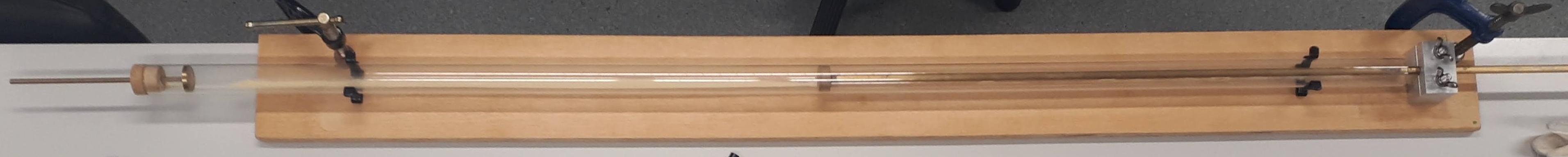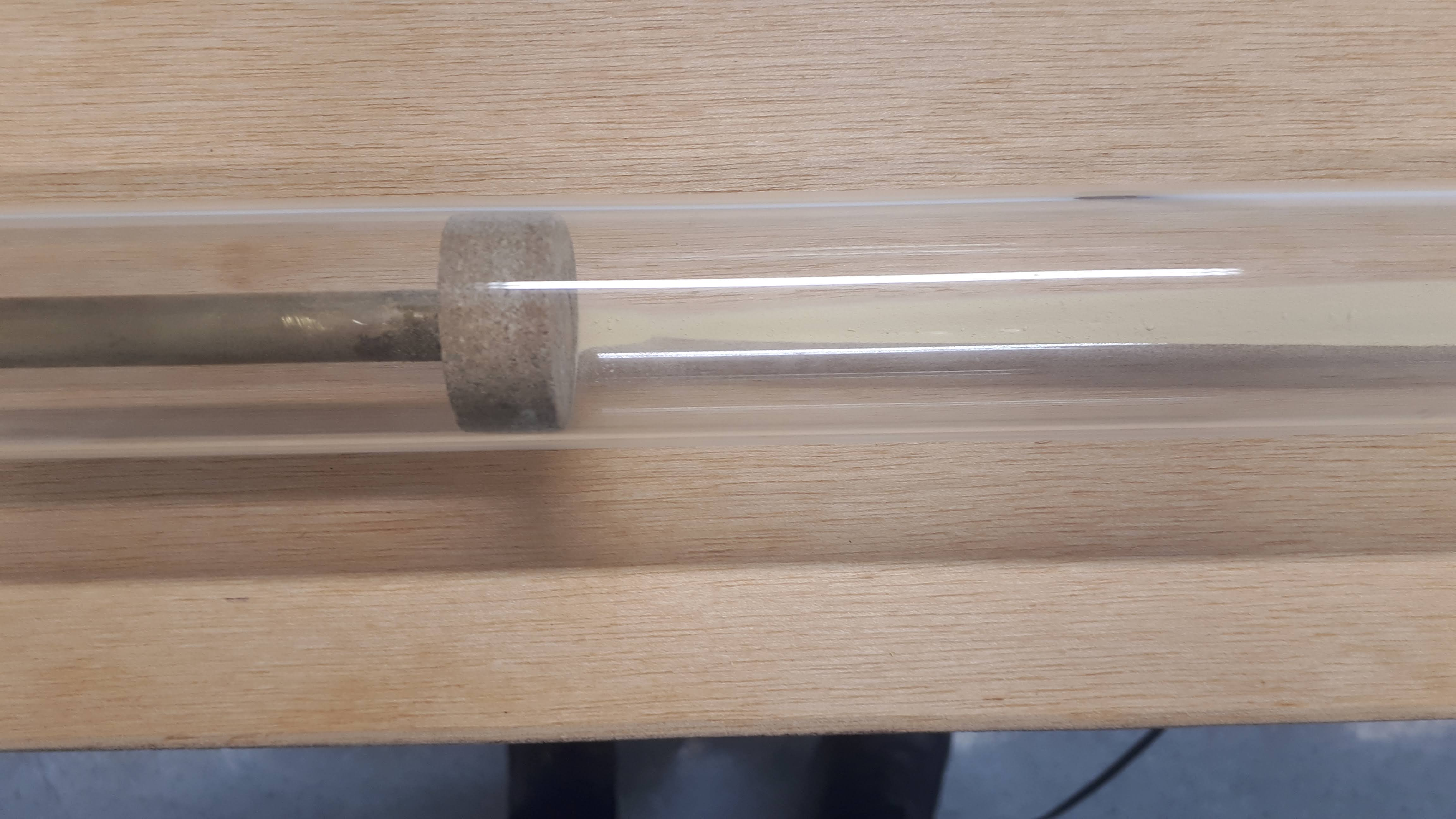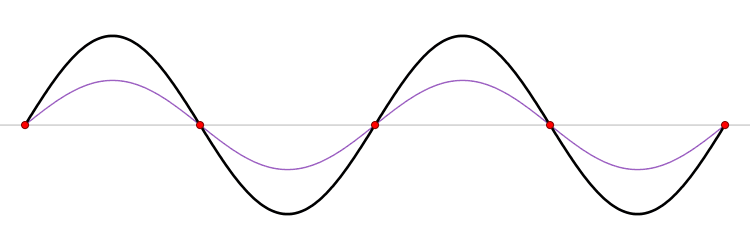# Kundts Tube

As discussed in previous experiments a spring returns to its original shape when the applied force that compresses or stretches it is removed. In fact, all materials are distorted when they are squeezed or stretched and many materials return to their original shape when the squeezing or stretching is removed. Such materials are termed elastic and this behaviour has its origin in the forces between atoms in the body. The inter-atomic forces that hold a solid together are particularly strong and it is necessary to apply a reasonable force in order to stretch or compress a solid object.

For the description of the elastic properties of linear objects like wires, rods, columns which are either stretched or compressed in only one direction, a parameter called the Young's modulus of the material. Young’s modulus is a measure of the ability of a material to withstand changes in length when under lengthwise tension or compression.

However an object can be deformed in ways other than stretching and compressing. Shearing forces are forces pushing one part of a body in one specific direction, and another part of the body in the opposite direction. One example is if we consider a solid rod with equal but opposite torques applied to at the two ends.

To investigate these properties in a brass rod we use a piece of equipment called Kundts Tube. The apparatus consists of a glass tube, inside which is lycopodium powder, supported on a wooden base as shown below. A clamp (the grey metal block on the right) holds a brass rod which has a cork disk attached to one end. the brass rod is clamped at it's centre point and so that the bar and disk extends inside the glass tube. A stopper and a smaller bar and metal disk closes the other end of the tube. This smaller rod and disc allow adjustment ot the size of the space between each metal disk without unclamping the larger brass bar. The whole apparatus is tightly clamped on a table, so that there will be no jerks on the tube during the experiment.This experiment is split into four parts:

Part 1: Determining the fundamental frequency of a brass bar when stretching

By pulling a cloth containing resin longitudinally along the brass rod set it into vibration. A microphone is used to pick up the sound produced and the frequency of the sound can be determined usng a fast fourier transform.

Part 2: Calcuation the speed of sound in the bar and hence Youngs Modulus

The frequency the bar vibrates at is dependant on it's length. Since we know the length of th bar and the fact that it is clamped in the middle , the wavelength of the vibrations can be determined. Using the measured frequency and the wavelength of the vibrations above the speed of sound traveling in the bar can be measured using

v = fλ

where v is the speed of sound, f is the frequency and λ is the wavelength.

Similarly Youngs Modulus can be calcu;ated using

v = √(Y / ρ)

where v is the speed of sound, Y is Youngs Modulus and ρ is the density of the material the sound is travelling through, in this case brass.

Part 3: Determination of the shear modulus for brass

The shear modulus is determined by rubbing the cloth on the brass rod in a rotationalmanner and following the procedure in Part 2 to determine the fundamental frequency of vibration and, hence the speed of sound in brass.

v = √(η / ρ)

where v is the speed of sound, η is the shear modulus and ρ is the density of brass.

Part 4: Determine the ratio of the velocity of sound in brass to that in air.

The brass rod is pulled to cause it to vibrate, this causes the cork disk at the end of the bar to also vibrate backwards and forwards. The air inside the glass tube is set into forced vibrations. As the wave travel through the air column and is them reflected back a standing wave is produced.The nodes of the waves are the red points where the wave stays at the zero point.  The dust in the tube will jump up due to the wave at any point which is not the node. As such the dust settles at the nodes. This can be seen in this video. To measure the speed of sound in air the wavelength is determined by measuring the distance between successive nodes as seen in the dust powder in the tube. The distance between two successive nodes is λ/2. Using the equation v = fλ (as described above) and knowing the frequency from the earlier parts of the experiment, the speed of sound can be calculated.## Department of Physics

Roinn na Fisice

Room 213 (Physics Office), 2nd floor, Kane Science Building, University College Cork, Ireland.,

Top